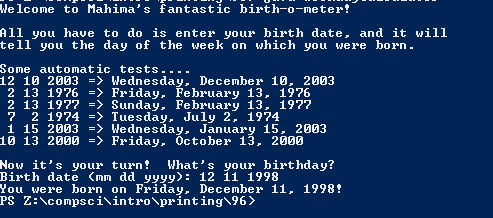# Assignment #96

## Code

```    /// Name: Mahima Choudhary
/// Period: 6
/// Program Name: Weekday Calculator
/// File Name: WeekdayCalculator.java
/// Date Finished: 3/28/2016

import java.util.Scanner;

public class WeekdayCalculator
{
public static void main( String[] args )
{
Scanner keyboard = new Scanner(System.in);

System.out.println("Welcome to Mahima's fantastic birth-o-meter!");
System.out.println();
System.out.println("All you have to do is enter your birth date, and it will");
System.out.println("tell you the day of the week on which you were born.");
System.out.println();
System.out.println("Some automatic tests....");
System.out.println("12 10 2003 => " + weekday(12,10,2003));
System.out.println(" 2 13 1976 => " + weekday(2,13,1976));
System.out.println(" 2 13 1977 => " + weekday(2,13,1977));
System.out.println(" 7  2 1974 => " + weekday(7,2,1974));
System.out.println(" 1 15 2003 => " + weekday(1,15,2003));
System.out.println("10 13 2000 => " + weekday(10,13,2000));
System.out.println();

System.out.print("Birth date (mm dd yyyy): ");
int mm = keyboard.nextInt();
int dd = keyboard.nextInt();
int yyyy = keyboard.nextInt();

// put a function call for weekday() here
System.out.println("You were born on " + weekday( mm, dd, yyyy) + "!");
}

public static String weekday( int mm, int dd, int yyyy )
{
int yy, total;
String date = "";

// bunch of calculations go here
yy = yyyy - 1900;
total = (yy/4) + yy + dd;
total = monthOffset(mm) + total;

if (isLeap(yyyy) == true)
{
if (mm == 1 || mm == 2)
{
total--;
}
}

date = weekday_name(total % 7) + ", " + monthName(mm) + " " + dd + ", " + yyyy;

return date;
}

// paste your functions from MonthName, WeekdayName, and MonthOffset here
public static String monthName( int month )
{
String result;

if (month == 1)
result = "January";
else if (month == 2)
result = "February";
else if (month == 3)
result = "March";
else if (month == 4)
result = "April";
else if (month == 5)
result = "May";
else if (month == 6)
result = "June";
else if (month == 7)
result = "July";
else if (month == 8)
result = "August";
else if (month == 9)
result = "September";
else if (month == 10)
result = "October";
else if (month == 11)
result = "November";
else if (month == 12)
result = "December";
else
result = "ERROR";

return result;
}

public static int monthOffset( int month )
{
int result;

if (month == 1)
result = 1;
else if (month == 2)
result = 4;
else if (month == 3)
result = 4;
else if (month == 4)
result = 0;
else if (month == 5)
result = 2;
else if (month == 6)
result = 5;
else if (month == 7)
result = 0;
else if (month == 8)
result = 3;
else if (month == 9)
result = 6;
else if (month == 10)
result = 1;
else if (month == 11)
result = 4;
else if (month == 12)
result = 6;
else
result = -1;

return result;
}

public static String weekday_name( int weekday )
{
String result = "";

if ( weekday == 1 )
{
result = "Sunday";
}
else if ( weekday == 2 )
{
result = "Monday";
}
else if ( weekday == 3 )
{
result = "Tuesday";
}
else if ( weekday == 4 )
{
result = "Wednesday";
}
else if ( weekday == 5 )
{
result = "Thursday";
}
else if ( weekday == 6 )
{
result = "Friday";
}
else if ( weekday == 7 )
{
result = "Saturday";
}
else if ( weekday == 0 )
{
result = "Saturday";
}
else
{
result = "error";
}

return result;
}

public static boolean isLeap( int year )
{
// years which are evenly divisible by 4 are leap years,
// but years divisible by 100 are not leap years,
// though years divisible by 400 are leap years
boolean result;

if ( year%400 == 0 )
result = true;
else if ( year%100 == 0 )
result = false;
else if ( year%4 == 0 )
result = true;
else
result = false;

return result;
}
}
```

### Picture of the output